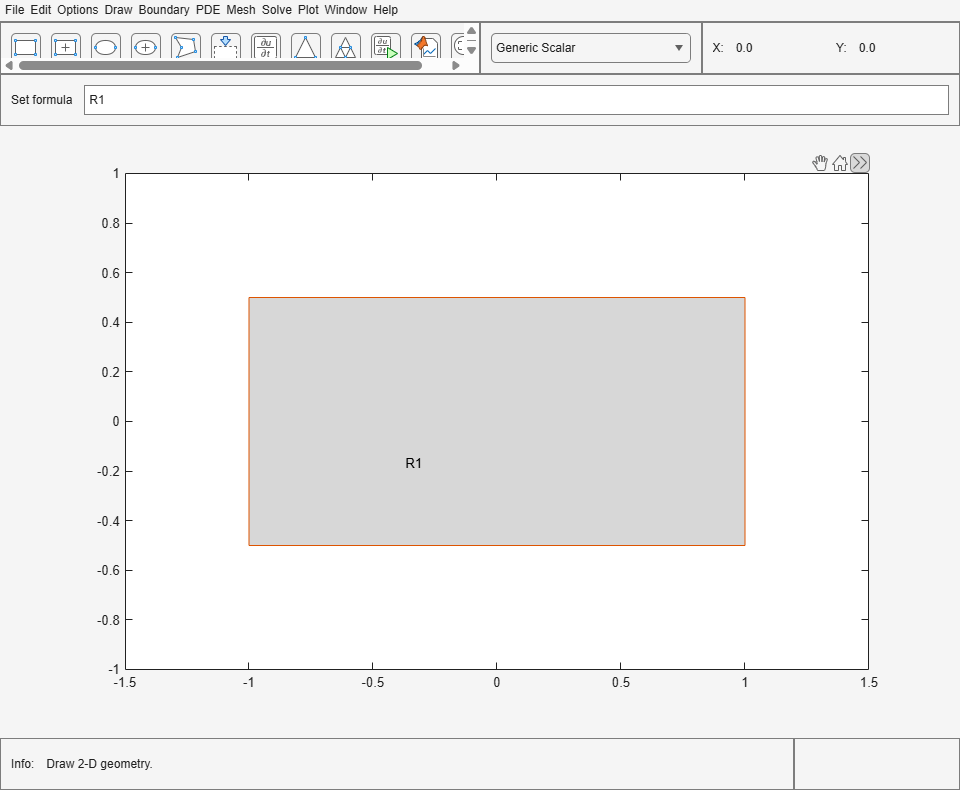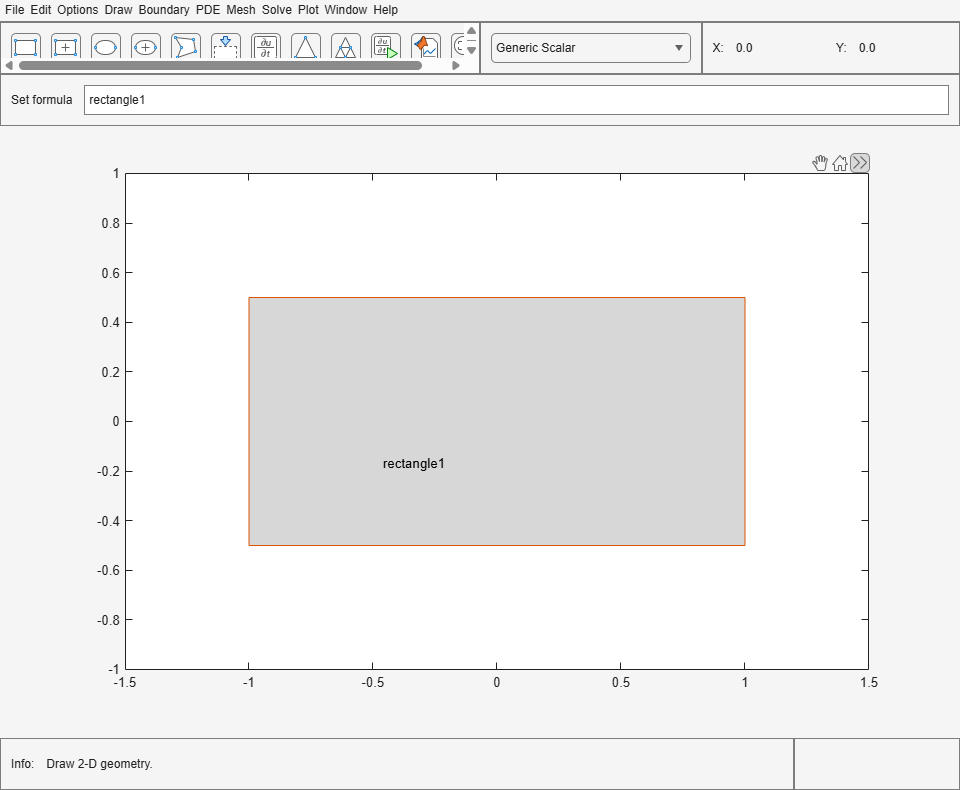# pderect

Draw rectangle in PDE Modeler app

## Syntax

``pderect([xmin xmax ymin ymax])``
``pderect([xmin xmax ymin ymax], label)``

## Description

example

````pderect([xmin xmax ymin ymax])` draws a rectangle with the corner coordinates defined by ```[xmin xmax ymin ymax]```. The `pderect` command opens the PDE Modeler app with the specified rectangle drawn in it. If the app is already open, `pderect` adds the specified rectangle to the app window without deleting any existing shapes.`pderect` updates the state of the geometry description matrix inside the PDE Modeler app to include the rectangle. You can export the geometry description matrix from the PDE Modeler app to the MATLAB® Workspace by selecting DrawExport Geometry Description, Set Formula, Labels.... For details on the format of the geometry description matrix, see `decsg`.```

example

````pderect([xmin xmax ymin ymax], label)` assigns a name to the rectangle. Otherwise, `pderect` uses a default name, such as `R1`, `R2`, and so on. For squares, `pderect` uses the default names `SQ1`, `SQ2`, and so on.```

## Examples

collapse all

Open the PDE Modeler app window containing a rectangle with the corners at `(-1,-0.5)`, `(-1,0.5)`, `(1,0.5)`, and `(1,-0.5)`.

`pderect([-1 1 -0.5 0.5])`

Call the `pderect` command again to draw a square with the corners at `(-0.25,-0.25)`, `(-0.25,0.25)`, `(0.25,0.25)`, and `(0.25,-0.25)`. The `pderect` command adds the square to the app window without deleting the rectangle.

`pderect([-0.25 0.25 -0.25 0.25])`Open the PDE Modeler app window and draw a rectangle with the corners at `(-1,-0.5)`, `(-1,0.5)`, `(1,0.5)`, and `(1,-0.5)`. Assign the name `rectangle1` to this rectangle.

`pderect([-1 1 -0.5 0.5],'rectangle1')`## Input Arguments

collapse all

Corner coordinates defining the rectangle, specified as a vector of real numbers.

Example: `pderect([-1 0 -1 0])`

Data Types: `double`

Name of the rectangle, specified as a character vector or string scalar.

Data Types: `char` | `string`

## Tips

• `pderect` opens the PDE Modeler app and draws a rectangle. If, instead, you want to draw rectangles in a MATLAB figure, use the `rectangle` function, for example, `rectangle('Position',[1,2,5,6])`.